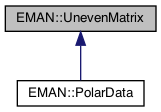EMAN2
EMAN::UnevenMatrix Class Reference

a general data structure for a matrix with variable x dim size for different y More...

`#include <polardata.h>`

Inheritance diagram for EMAN::UnevenMatrix:[legend]

## Classes

struct  Xdim
struct to define x dimension size for each y, x0 is inclusive, x1 is one after the maximum, [x0, x1), so the corresponding x dim size is (x1-x0) More...

## Public Member Functions

UnevenMatrix ()

virtual ~UnevenMatrix ()

int get_xsize (int y)
get the x dim size for a given y More...

int get_xmin (int y)
get the minimal x dim value for a given y More...

int get_xmax (int y)
get the maximal x dim value for a given y, note: x1 is one out of the max More...

int get_size ()
get the total size of the data block More...

## Protected Member Functions

void alloc_data ()
allocation memory for data array More...

## Protected Attributes

float * data
store all data in one dimension float array for cache efficiency, we calculate the offset for x, y dimension More...

map< int, Xdimdesc_data
describe for each y, the x dimension's size and range More...

int tot_size
the total size of the data More...

## Detailed Description

a general data structure for a matrix with variable x dim size for different y

Definition at line 49 of file polardata.h.

## ◆ UnevenMatrix()

 EMAN::UnevenMatrix::UnevenMatrix ( )
inline

Definition at line 52 of file polardata.h.

52 : data(0) {
53 printf("Welcome to UnevenMatrix\n");
54 }
float * data
store all data in one dimension float array for cache efficiency, we calculate the offset for x,...
Definition: polardata.h:123

## ◆ ~UnevenMatrix()

 virtual EMAN::UnevenMatrix::~UnevenMatrix ( )
inlinevirtual

Definition at line 55 of file polardata.h.

55 {
56 if(data) {
57 delete data;
58 data = 0;
59 }
60 printf("Destructor of UnevenMatrix...\n");
61 }

References data.

## ◆ alloc_data()

 void UnevenMatrix::alloc_data ( )
protected

allocation memory for data array

Returns
int
Exceptions
 InvalidValueException if the desc_data map size is zero

Definition at line 55 of file polardata.cpp.

56{
57 if( desc_data.size() == 0 ) {
58 LOGERR("No data space need to be allocated for UnevenMatrix, check you desc_data...");
59 throw InvalidValueException((float)desc_data.size(), "desc_data size == 0");
60 }
61
62 int size = 0; //total number of float need to be stored in data block
63 map< int, Xdim >::const_iterator iter;
64 int y = 0;
65 for( iter = desc_data.begin(); iter != desc_data.end(); ++iter ) {
66 y = (*iter).first;
67 size += get_xsize(y);
68 }
69
70 this->tot_size = size;
71 try {
72 this->data = new float[size];
73 }
74 catch( bad_alloc exception ) {
75 LOGERR("memory allocation for UnevenMatrix failed");
76 }
77 catch(...) {
78 LOGERR("Unknown error in memory allocation for UnevenMatrix");
79 }
80}
int tot_size
the total size of the data
Definition: polardata.h:129
map< int, Xdim > desc_data
describe for each y, the x dimension's size and range
Definition: polardata.h:126
int get_xsize(int y)
get the x dim size for a given y
Definition: polardata.h:66
#define InvalidValueException(val, desc)
Definition: exception.h:285
#define LOGERR
Definition: log.h:51
#define y(i, j)
Definition: projector.cpp:1516

References data, desc_data, get_xsize(), InvalidValueException, LOGERR, tot_size, and y.

## ◆ get_size()

 int EMAN::UnevenMatrix::get_size ( )
inline

get the total size of the data block

Returns
int the number of float stored in data

Definition at line 92 of file polardata.h.

92 {
94 }

References tot_size.

## ◆ get_xmax()

 int EMAN::UnevenMatrix::get_xmax ( int y )
inline

get the maximal x dim value for a given y, note: x1 is one out of the max

Parameters
 y int the y value for which we need the corresponding maximal x dim value
Returns
int the maximal x dim value for a given y

Definition at line 86 of file polardata.h.

86 {
87 return desc_data[y].x1 - 1;
88 }

References desc_data, and y.

## ◆ get_xmin()

 int EMAN::UnevenMatrix::get_xmin ( int y )
inline

get the minimal x dim value for a given y

Parameters
 y int the y value for which we need the corresponding minimal x dim value
Returns
int the minimal x dim value for a given y

Definition at line 79 of file polardata.h.

79 {
80 return desc_data[y].x0;
81 }

References desc_data, and y.

## ◆ get_xsize()

 int EMAN::UnevenMatrix::get_xsize ( int y )
inline

get the x dim size for a given y

Parameters
 y int the y value for which we need the x dim size
Returns
in tthe x dim size

Definition at line 66 of file polardata.h.

66 {
67 int xsize = desc_data[y].x1 - desc_data[y].x0;
68 if( xsize <= 0 ) {
69 throw InvalidValueException(xsize, "xsize <= 0");
70 }
71 else {
72 return xsize;
73 }
74 }

References desc_data, InvalidValueException, and y.

Referenced by alloc_data().

## ◆ data

 float* EMAN::UnevenMatrix::data
protected

store all data in one dimension float array for cache efficiency, we calculate the offset for x, y dimension

Definition at line 123 of file polardata.h.

Referenced by alloc_data(), and ~UnevenMatrix().

## ◆ desc_data

 map< int, Xdim > EMAN::UnevenMatrix::desc_data
protected

describe for each y, the x dimension's size and range

Definition at line 126 of file polardata.h.

Referenced by alloc_data(), get_xmax(), get_xmin(), and get_xsize().

## ◆ tot_size

 int EMAN::UnevenMatrix::tot_size
protected

the total size of the data

Definition at line 129 of file polardata.h.

Referenced by alloc_data(), and get_size().

The documentation for this class was generated from the following files: